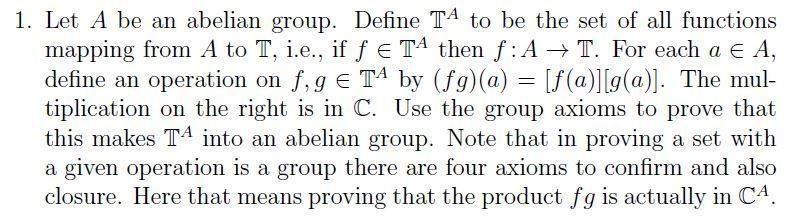# Prove a group is abelian

## Homework Statement## The Attempt at a Solution

For the first question, since [f(a)][g(a)] is in C, can I just say that since C is a ring, it is an abelian group, then the four axioms are proven? Then just show closure? Probably not I'm guessing. Associativity of multiplying functions seems so fundamental to me that I really don't know what to do...

For the second question, shall I start by using composition of functions and then the properties of composition of a function and its inverse, and then go on about the identity function?

#### Attachments

Last edited by a moderator:

## Answers and Replies

I'd say question one is asking you to flesh out the group structure.

1. What's the identity element? (make sure it's a member of C^A).

2. For each element f, what's the inverse element? (make sure it's well defined
- namely, why can you find f^-1 for each f? )

Closure is trivial but should be mentioned, namely why is (fg) a well
defined member of C^A when f and g are?

Associativity (and Abelian-ness) of course follow from the properties of C,
but you should have a proof showing f(gh) =(fg)h.

For question 2. I don't know what A-hat is. What do you know
about group homomorphisms at this point?